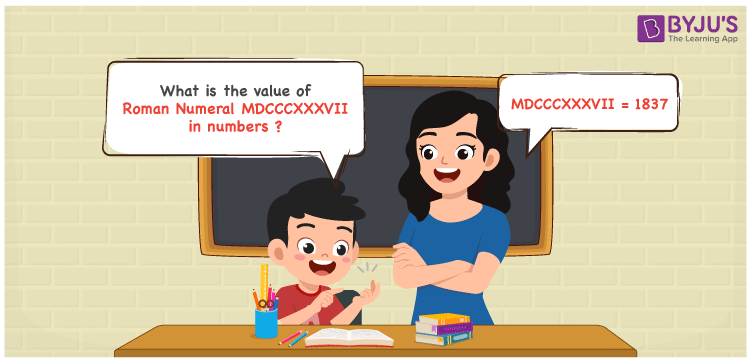Checkout JEE MAINS 2022 Question Paper Analysis : Checkout JEE MAINS 2022 Question Paper Analysis :

# MDCCCXXXVII Roman Numerals

MDCCCXXXVII Roman Numerals is 1837. In this article, steps used to write MDCCCXXXVII in numeric form are provided in an easy to understand language. Learn the roman numerals conversion effectively and increase confidence to answer questions of varying difficulty levels. Therefore, the roman numerals MDCCCXXXVII is written as 1837 in numbers.

 Number Roman Numeral 1837 MDCCCXXXVII## How to Write MDCCCXXXVII Roman Numerals in Numbers?

This section has all the details necessary for the students to understand the conversion of MDCCCXXXVII to numbers.

MDCCCXXXVII = M + D + C + C + C + X + X + X + V + I + I

MDCCCXXXVII = 1000 + 500 + 100 + 100 + 100 + 10 + 10 + 10 + 5 + 1 + 1

MDCCCXXXVII = 1837

## Video Lesson on Roman Numerals## Frequently Asked Questions on MDCCCXXXVII Roman Numerals

### Why is MDCCCXXXVII the roman numerals of 1837?

We know that;

M is the roman numerals of 1000

DCCC is the roman numerals of 800

XXX is the roman numerals of 30

VII is the roman numerals of 7

So MDCCCXXXVII is the roman numeral of 1837.

### How can we write 1900 – 63?

We know that

1900 – 63 = 1837

Hence, 1900 – 63 is 1837 which is written as MDCCCXXXVII.

### Find the remainder if MDCCCXXXVII is divided by IX.

We know that

MDCCCXXXVII = 1837

IX = 9

The remainder if MDCCCXXXVII is divided by IX is I.